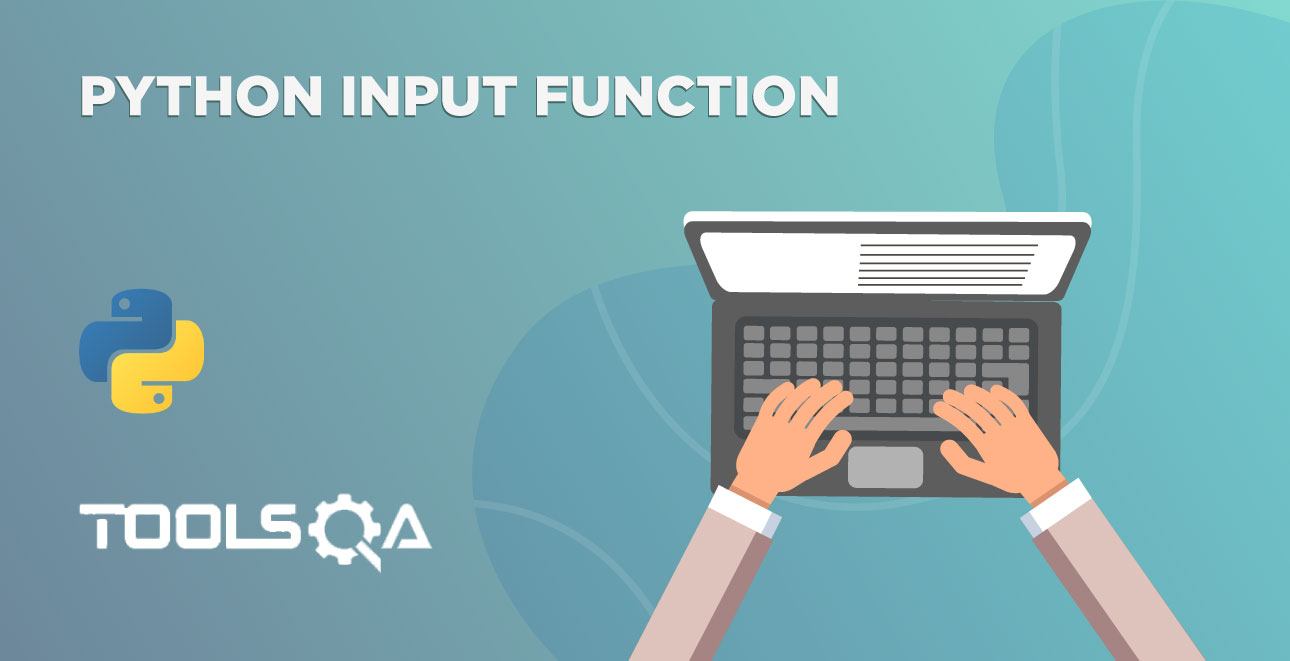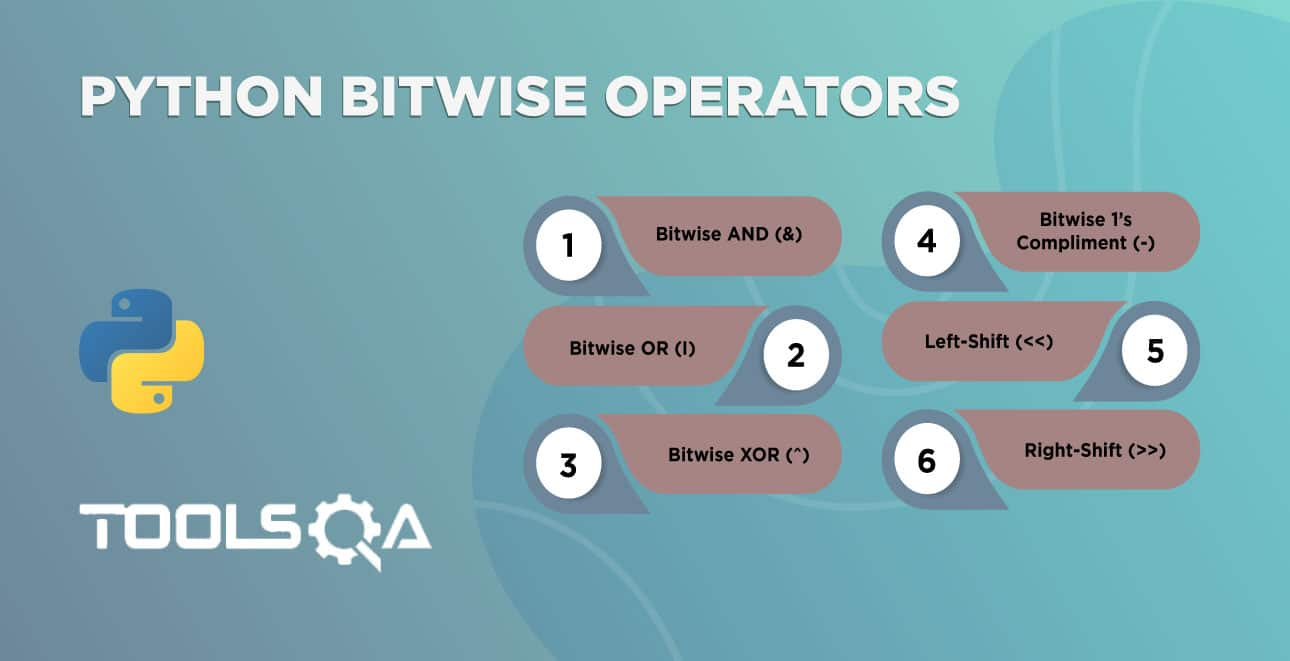We have seen how to perform arithmetic operations on integer and floats. We can use `+` and `*` operators on a string as well. However, it behaves differently. Let's have a quick look at various types of Python String Operator :

• First is Python String Operator - Concatenation Operator
• Second is Python String Operator - Replication Operator

## Python String Operator - Concatenation Operator

The addition operator + is also known as a concatenation operator when applied on two python strings.

What is concatenation? The meaning of the word concatenation tells everything about the functionality. It links in a chain or series. In other words, the concatenation operator connects one string at the end of the other.

For example, let's have a look at some expressions:

• "Tools" + "QA" => "ToolsQA"
• "Hi, " + "I'm from ToolsQA" => "Hi, I'm from ToolsQA"
• "Learning " + "Python" => "Learning Python"

The general expression of the concatenation operators is.

``````string + string
``````

Let's get into the coding.

``````# concatenation two strings
name = "Tools" + "QA"
print(name)
``````Subsequently, run the code and compare the result with the expected output.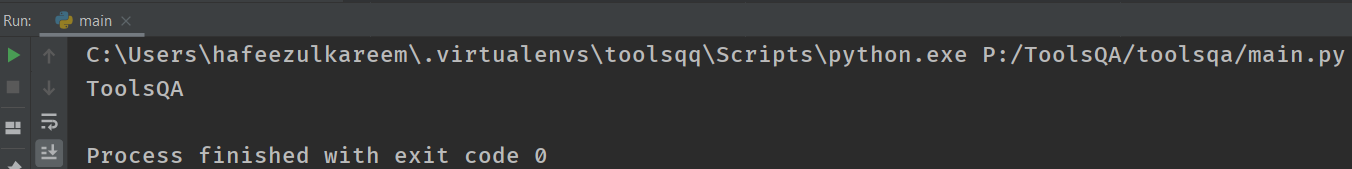If you look at the output, the addition of the string "QA" happened at the end of the string "Tools." Yeah! We got what we have expected.

We know that both expressions 2 + 3 and 3 + 2 produces the same result when we do the arithmetic operation. What about "Tools" + "QA" and "QA" + "Tools"? Did they give the same output? No! We don't get the same output. Let's see why.

• Firstly, Concatenation operators join the second operand (string) at the end of the first operand (string).
• Therefore, the expression "Tools" + "QA" gives "ToolsQA" whereas the expression "QA" + "Tools" gives "QATools".
• And the results are not the same.
• Moreover, the arithmetic addition operator is commutative while string concatenation is not.

Let's see it by executing the following code.

``````# String concatenation is not commutative
name_one = "Tools" + "QA"
name_two = "QA" + "Tools"
print(name_one)
print(name_two)
``````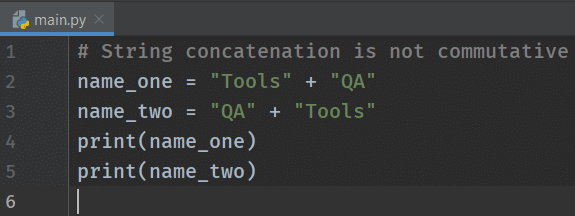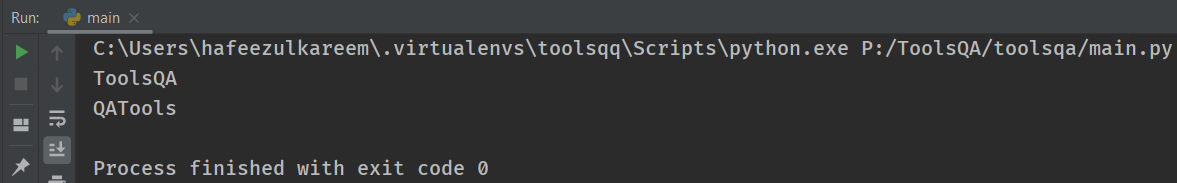We can see that they are not the same.

Remember, the + operators act as a concatenator only if both the operands are strings. Additionally, if anyone of them is not a string, then we will get an error as we have seen in the previous tutorials. Let's recap it by executing an example.

``````# concatenation
name = "ToolsQA" + 2020
print(name)
``````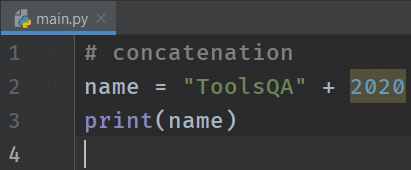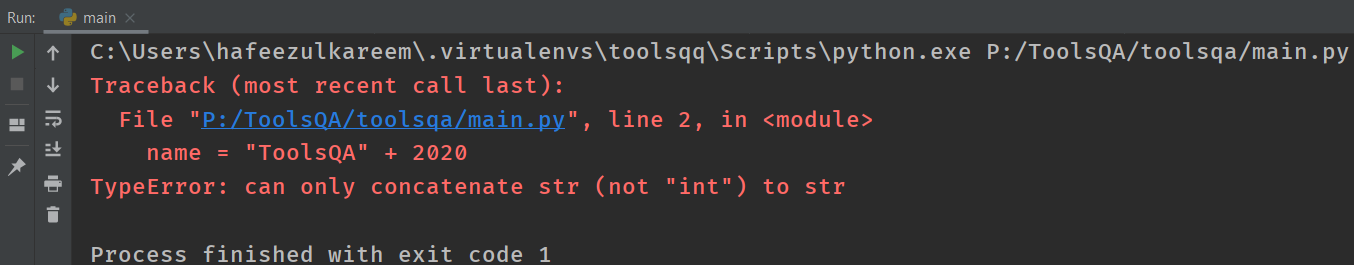Got an error, as we expected.

Do you think we can use the concatenation operator directly in print() function? Yeah! We can. We have already used it in the previous tutorials. For example, see the below:

``````# string concatenation in print
print("Tools" + "QA")
``````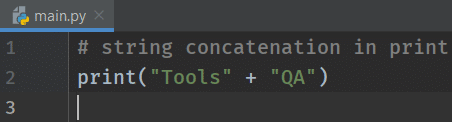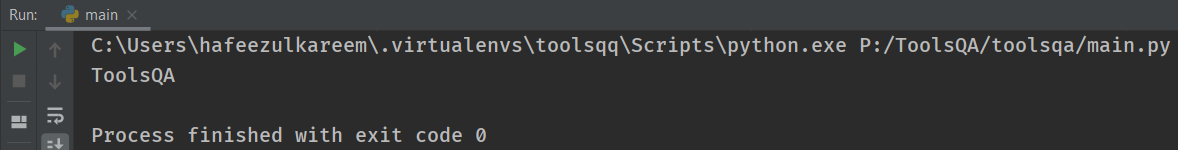We can use concatenation in print () function.

Note:- If we pass an expression to the print() function, then it gives the output after evaluating that expression.

## Python String Operator - Replication Operator

The multiplication operator acts as a replication operator when we have one string and one integer as operands.

What is replication? First, understand the meaning of the word replication. The purpose is to make an exact copy. Moreover, the replication operator produces the n number of copies of a string, n may be any integer.

We can use the replication operator as follows in general.

``````string * integer
integer * string
``````

Those are the two general formats of the * operator with strings. We will get the same result in both formats. Let's check it with the following example.

``````# replication operator
print("ToolsQA" * 2)
print(2 * "ToolsQA")
``````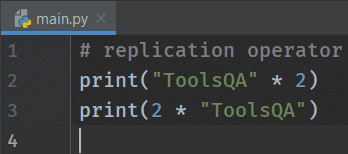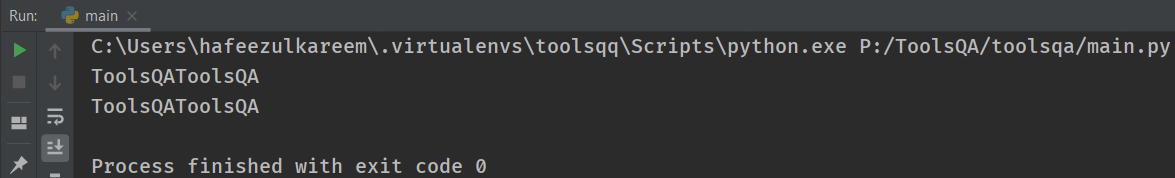If you see the output of the code, we got the same result for both expressions. Additionally, let's examine the following examples to understand how the replication operator works.

• "ToolsQA" * 3 => "ToolsQAToolsQAToolsQA"
• 3 * "Hi" => "HiHiHi"
• "7" * 3 => "777"

Let's code.

``````# replication
print("ToolsQA" * 3)
print(3 * "Hi")
print("7" * 3)
``````Run the code and compare the result.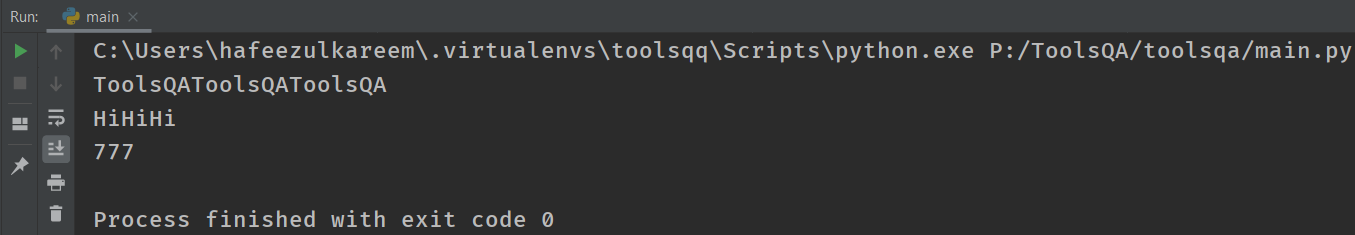We got the expected output.

What is the output for the expression "ToolsQA" **** 0*** and "ToolsQA" * -1? Execute the following code, see the output.

``````# replication
print("ToolsQA" * 0)
print("ToolsQA" * -1)
``````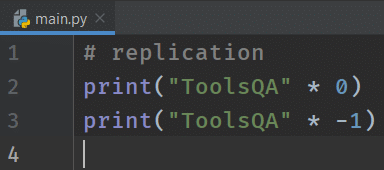We got nothing in the output console. What happened? We will get empty strings if we pass a number which less than or equal to 0. Hence we got two lines as output.

What if we usefloats in place of integers? Let's check it with the following code.

``````# replication
print("ToolsQA" * 1.0)
``````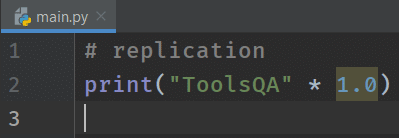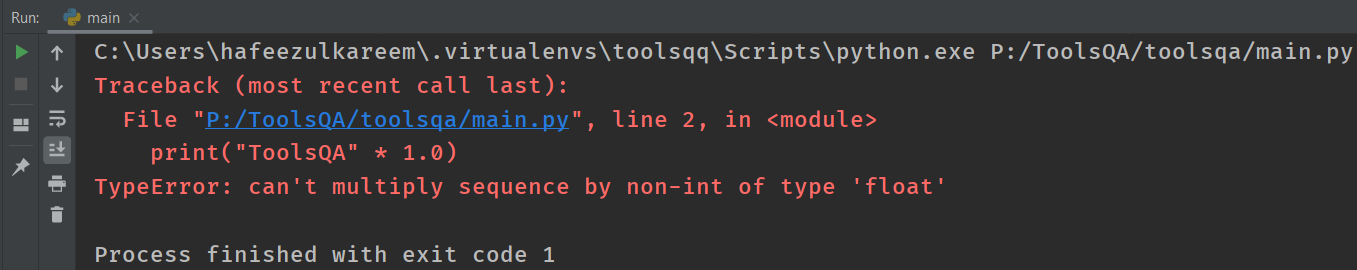We can't use floats in the replication operation. Since we got an error. We must use integers in the replication operations.

What if we pass two string arguments to the replication operator? Think about it and check it with the following code.

``````# replication
print("ToolsQA" * "Hi")
``````

What happens if you use replication operator by converting a string to a number? Will we get the same results as before?

Let's see with the following examples.

Run the following example without str to int conversion and see what we get.

``````# replication operator
num_str = "5"

# without converting: str -> int
# using replication operator
print(num_str * 3)

``````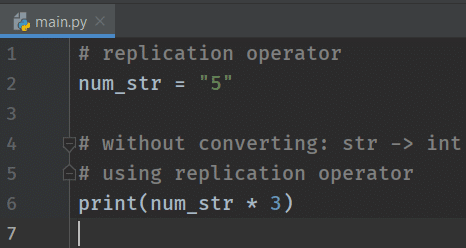Consequently, we got the output as we expected with the replication operator. Execute to see the output of the following example.

``````# replication operator
num_str = "5"

# converting: str -> int
# using replication operator
print(int(num_str) * 3)
``````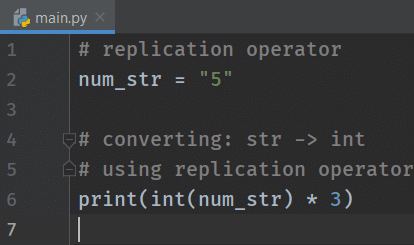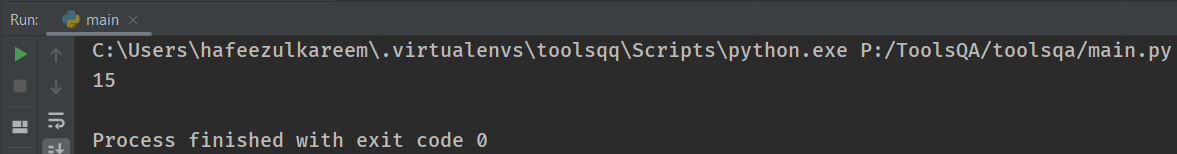We got 15. Did you expect that? We got 15 as output as * works as a multiplication operator with two integer operands.

Moreover, we have converted the string to an integer before using the * operator. The * operator then becomes a multiplication operator instead of a replication operator.

To conclude, that's it about the string operators. While you would not use the replication operator frequently, there will be heavy use of the concatenation operator in coding.

## Key Takeaways

• We can use + operator as a concatenation operator with two string operands.
• Moreover, All Operands must be strings in concatenation operation. E.g. "Hi" + "Tools" + "QA" = HiToolsQA
• The concatenation operation is not commutative. That is to say, "Hi" + "ToolsQA" and "ToolsQA" + "Hi" produce two different outputs.
• ** is considered as a replication operator with one string and one integer operands.*
• Additionally, we can use string or integer as the first operand and vice-versa. And we will get the same results with both formats. For example, "Hi" * 3 and 3 * "Hi"  both will produce "HiHiHi."
• We will get the error if we use any data type other than an integer in replication operators.
• Moreover, using a replication operator with a number less than or equal to 0  will give an empty result. For example, "Hi" * 0, -7 * "Hi", etc.
• Lastly, the replication operator behaves as a multiplication operator if both operands are integers.

## Practice Yourself

``````#Problem 1
# input:- ToolsQA
print("Hi, " + input("Enter your name:- "))
``````
``````#Problem 2
# input:- ToolsQA
print(input("Enter your name:- ") + " That's it!" * 3)
``````
``````#Problem 3
# input:- ToolsQA
print(input("Enter your name:- ") * 3 + " That's it!")
``````
``````#Problem 4
# Follow the instructions to write a program
# 1. Initialize a variable with number 3
# 2. Initialize a string with *
# 3. Now, print the string (2 * number - 1) times using replication operator
# 4. Repeat the 3rd step for 4 more times. Total 5 rows should be printed
``````
``````#Problem 5
# Write a program to get the following pattern as output
# There are 10 hyphens (-) on top and bottom of the rectangle
# We have 5 pipes (|) in on right and left of the rectangle
# Try it yourself to get familiar with the string operators

``````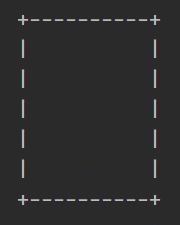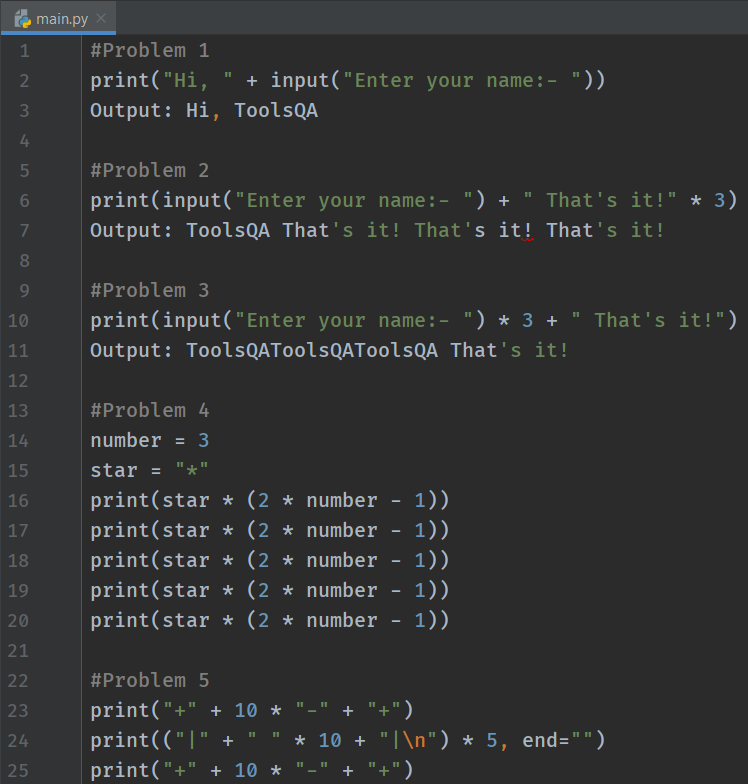## What Next?

Congratulations! We have completed two modules of the course. Additionally, we learned a lot in Python World. However, there is more to come. Challenge yourself with the practice problems.

Conclusively, we will now move into the third module of the course from the next tutorial. We will learn about conditionals, loops, flow control, data collections, and different sets of operators. Moreover, the programs and concepts will get more complicated from here, but don't worry. We will take you to step by step on every single thought. You just need to ensure you keep practicing!

Happy Coding :)